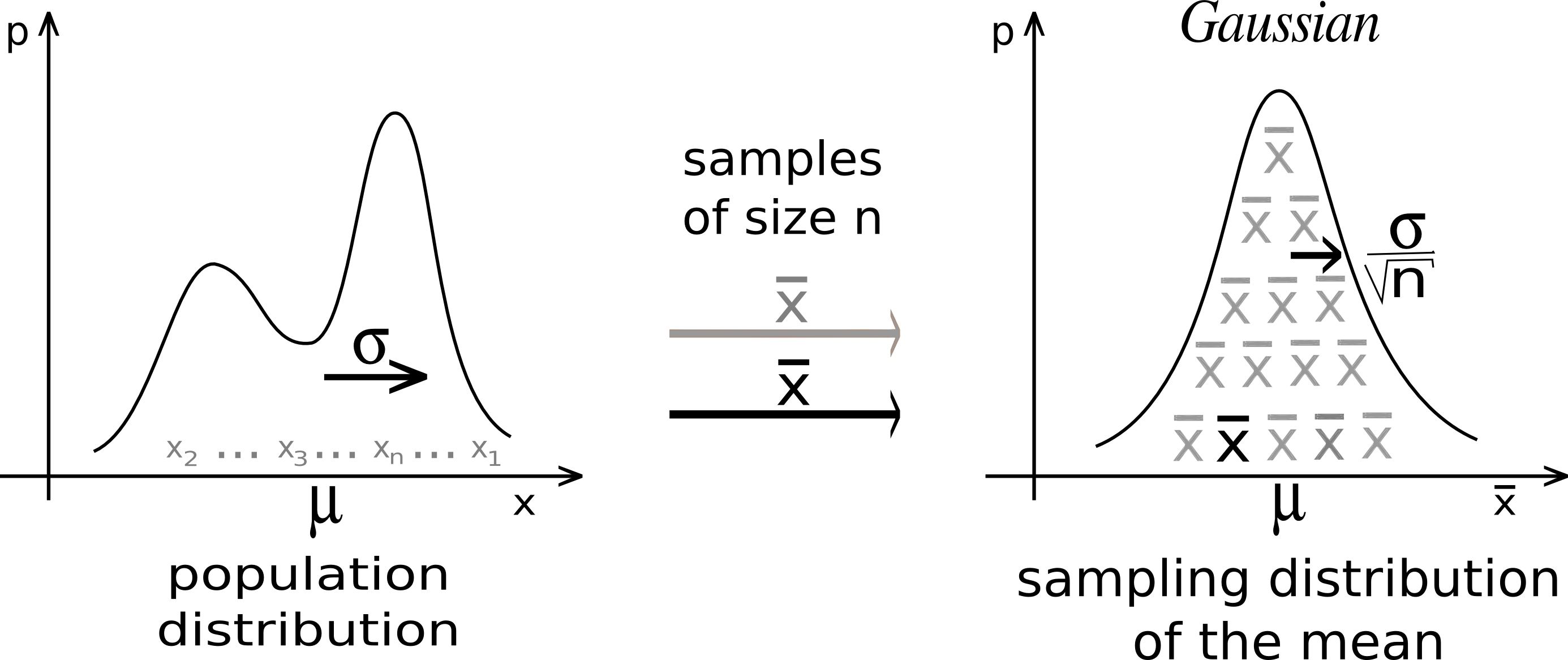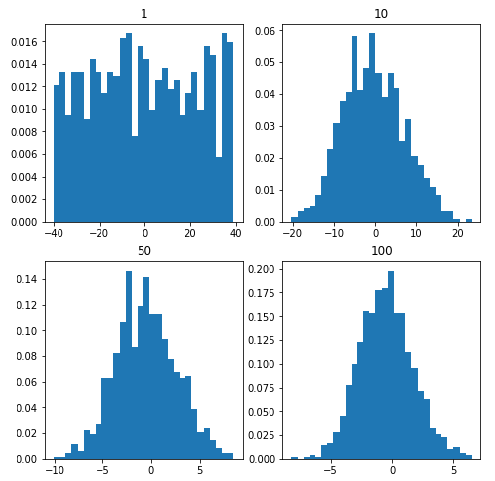# Python – Central Limit Theorem

• Difficulty Level : Hard
• Last Updated : 29 May, 2021

The definition:

The sample mean will approximately be normally distributed for large sample sizes, regardless of the distribution from which we are sampling.

Attention reader! Don’t stop learning now. Get hold of all the important Machine Learning Concepts with the Machine Learning Foundation Course at a student-friendly price and become industry ready.Suppose we are sampling from a population with a finite mean and a finite standard-deviation(sigma). Then Mean and standard deviation of the sampling distribution of the sample mean can be given as:Whererepresents the sampling distribution of the sample mean of size n each,andare the mean and standard deviation of the population respectively.
The distribution of the sample tends towards the normal distribution as the sample size increases.
Code: Python implementation of the Central Limit Theorem

## python3

 import numpyimport matplotlib.pyplot as plt # number of samplenum = [1, 10, 50, 100] # list of sample meansmeans = []  # Generating 1, 10, 30, 100 random numbers from -40 to 40# taking their mean and appending it to list means.for j in num:    # Generating seed so that we can get same result    # every time the loop is run...    numpy.random.seed(1)    x = [numpy.mean(        numpy.random.randint(            -40, 40, j)) for _i in range(1000)]    means.append(x)k = 0 # plotting all the means in one figurefig, ax = plt.subplots(2, 2, figsize =(8, 8))for i in range(0, 2):    for j in range(0, 2):        # Histogram for each x stored in means        ax[i, j].hist(means[k], 10, density = True)        ax[i, j].set_title(label = num[k])        k = k + 1 plt.show()

Output:It is evident from the graphs that as we keep on increasing the sample size from 1 to 100 the histogram tends to take the shape of a normal distribution.
Rule of thumb:
Of course, the term “large” is relative. Roughly, the more “abnormal” the basic distribution, the larger n must be for normal approximations to work well. The rule of thumb is that a sample size n of at least 30 will suffice.
Why is this important?
The answer to this question is very simple, as we can often use well developed statistical inference procedures that are based on a normal distribution such as 68-95-99.7 rule and many others, even if we are sampling from a population that is not normal, provided we have a large sample size.

My Personal Notes arrow_drop_up